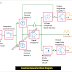Hey, in this article, I am going to explain the Function Generator Block Diagram. Here you can easily understand the working principle of a function generator from this Block Diagram of Function Generator. First of all, let's know what is a function generator? The function generator is an electronic device or circuit used to generate electronic signals with different waveforms and different frequencies. A function generator can generate a sine wave, triangular wave, or square wave electronic signals. Also, a function generator can generate electronic signals from a few hertz to megahertz frequencies. A function generator also provides the facility to vary the frequency and shape of the waveforms as per our requirements.

## Function Generator Block Diagram

Carefully see the block diagram of a function generator given below. You can easily understand the working of the function generator.

## Function Generator Working Principle

The above diagram describes a three-output function generator - Square Wave, Triangular Wave, and Sine Wave

Let's discuss each block of the circuit

### Frequency Control Knob

Using this frequency control knob we can adjust the frequency of the output waveforms as per our requirements. It is basically nothing but a potentiometer. Its main function is to vary the voltage to regulate the current source 1 and 2.

### Frequency Controlled Circuit

It consists of resistors and capacitors. As per the charging and discharging rates of the capacitor, it regulates the two current sources(1 and 2). Here, different types of network circuits are used such as RC networks.

### Current Sources 1 and 2

These are the constant current sources input to the signal integrator. Among these two current sources, source 1 provides a forward current supply, and source 2 provides a reverse current supply to the integrator. So using the current source 1 the signal integrator can create the positive half cycle of the waveform and using the current source 2 the signal integrator can create the negative half cycle of the waveform.

### Signal Integrator

Mainly the signal integrator is a current-to-voltage converter circuit. In normal, it takes the current from source 1 and generates a triangular waveform. The frequency of the triangular waveform depends upon the current supplied by the constant current supply sources.

### Voltage Comparator

The main function of the voltage comparator multivibrator is to control the magnitude or voltage level of the waveform. When the magnitude or voltage of the waveform reaches the maximum predetermined level in the positive half cycle, the voltage comparator switches the current sources means it cuts off source 1. Again when the voltage level reaches the maximum predetermined level in the negative half cycle, the voltage comparator switches the current source into source 1.

So, as the voltage comparator can control both the positive and negative half cycle of the waveform very precisely, it can generate the square wave electronic signal.

### Resistance Diode Shaping Circuit

It takes the triangular wave signal from the signal integrator as an input and generates the sine wave signal. This Resistance diode shaping network changes the slope of the triangular wave. So the amplitude continuously changes with time and produces a sinusoidal wave having less than 1% distortion.

Function Generator Block Diagram and Working PrincipleReviewed by Author on February 15, 2021 Rating: 5